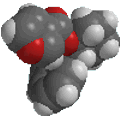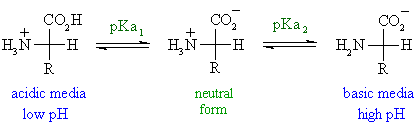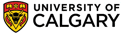Chapter 27: Amino Acids, Peptides and ProteinsIsoelectronic point, pI
• The isoelectronic point or isoionic point is the pH at which the amino acid does not migrate in an electric field.
• This means it is the pH at which the amino acid is neutral, i.e. the zwitterion form is dominant.
• A table of pKa and pI values can be found on the next page.
• The pI is given by the average of the pKas that involve the zwitterion, i.e. that give the boundaries to its existence.
There are 3 cases to consider....
• neutral side chains
These amino acids are characterised by two pKas : pKa1 and pKa2 for the carboxylic acid and the amine respectively.
The isoelectronic point will be halfway between, or the average of, these two pKas, i.e.   pI = 1/2 (pKa1 + pKa2). This is most readily appreciated when you realise that at very acidic pH (below pKa1) the amino acid will have an overall +ve charge and at very basic pH (above pKa2 ) the amino acid will have an overall -ve charge.  For the simplest amino acid, glycine, pKa1= 2.34 and pKa2 = 9.6, pI = 5.97.The other two cases introduce other ionisable groups in the side chain "R" described by a third acid dissociation constant, pKa3
• acidic side chains
The pI will be at a lower pH because the acidic side chain introduces an "extra" negative charge. So the neutral form exists under more acidic conditions when the extra -ve has been neutralised.  For example, for aspartic acid shown below, the neutral form is dominant between pH 1.88 and 3.65, pI is halfway between these two values, i.e.   pI = 1/2 (pKa1 + pKa3),  so pI = 2.77.• basic side chains
The pI will be at a higher pH because the basic side chain introduces an "extra" positive charge. So the neutral form exists under more basic conditions when the extra +ve has been neutralised.  For example, for histidine, which was discussed on the previous page, the neutral form is dominant between pH 6.00 and 9.17, pI is halfway between these two values, i.e.  pI = 1/2 (pKa2 + pKa3),  so pI = 7.59.© Dr. Ian Hunt, Department of Chemistry